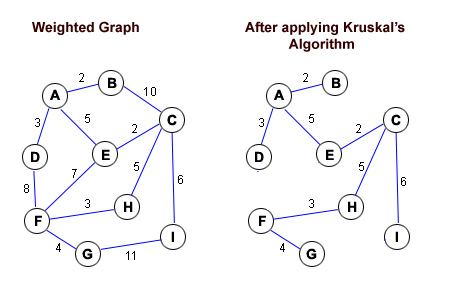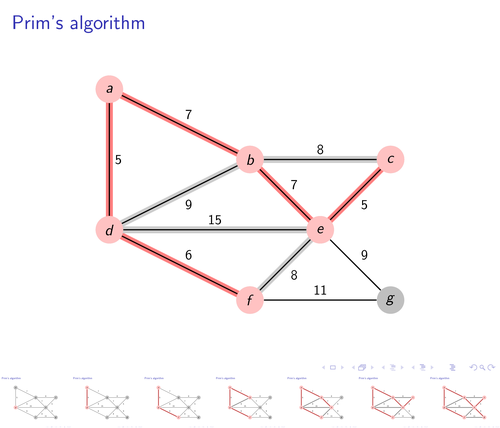# Difference between Kruskal and Prim

The only thing common between Kruskal and Prim is that they are computing algorithms. Apart from that, they are very different from each other.

The first difference is that Kruskal’s algorithm begins with an edge, on the other hand, Prim’s algorithm starts from a node.

Kruskal’s algorithm does not have to be on a connected graph, however, in Prim’s algorithm the graph must be connected.

The time complexity of Kruskal is O(logV), whereas, the time complexity of Prim’s algorithm is O(V2). Another major difference between the two is that Prim’s algorithm reaches from one node to the other, while this is not the case in Kruskal’s algorithm.

### Instructions

• 1

Kruskal’s Algorithm

This algorithm was created by Joseph Kruskal and made its appearance in the 1956 proceedings of the American Mathematical Society. This algorithm can be best described and explained in three simple steps.

If you have a graph with n nodes and also the weight of each edge then:

- The first step is the selection of the arc which consists of the least weight of the graph, and add to the tree, by deleting it from the graph.

- Now out of the remaining, the edge with the least weight is selected but such that it is not from the cycle. Now the edge needs to be added in the tree and deleted from graph.

- The third step involves you repeating the second step.

Here the algorithm continues selecting the edge on every cycle,  starting from the least weighted edge only.

Hence, in this algorithm the graph does not need to be connected.• 2

Prim’s Algorithm

Czech mathematician Vojtěch Jarník invented this algorithm in 1930 and later in 1957, it was developed further by Robert C. Prim who was a computer scientist. It was then rediscovered in 1959 by Edsger Dijkstra.

This algorithm can also be best understood in the following three steps:

In reference to a connected graph with n nodes and a given weight of every edge,

- The first step is to select any node right from the graph and add it in the tree which is to be the first node.

- Now select an edge with the minimum weight and which is also connected to the tree. The edge and the node need to be added at the other end of the tree after which remove the edge from the graph.

- You now need to repeat the second step till the time n-1 edges get added to the tree.

In this, the tree begins from a single node and then expands onwards on every cycle. Therefore, for the proper working of the algorithm it is vital that the graph is connected.•